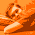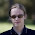## Monday, March 28, 2011

### Changing the sign of float values using SSE code

The IEEE 754 floating point format defines the memory layout for the `C++ float` datatype. It consists of a one bit sign, the 8 bit exponent and 23 bits that store the fractional part of the value.
```float x = [sign (1 bit) | exponent (8bit) | fraction (23bit)]
```
We can use this knowledge about the memory-layout in order to change the sign of floating point values without the need for floating point arithmetic. For example, calculating the absolute value of a floating point number is equivalent to setting the sign bit to zero. In SSE we can do this for four float values simultaneously by using a binary mask and logical operations:
```static const __m128 SIGNMASK =
_mm_castsi128_ps(_mm_set1_epi32(0x80000000));
__m128 val = /* some value */;
__m128 absval = _mm_andnot_ps(SIGNMASK, val); // absval = abs(val)
//...
```
In a similar way we can negate floating point numbers by simply negating their highest bit:
```__m128 val = /* some value */;
__m128 minusval = _mm_xor_ps(val, SIGNMASK); // minusval = -val
//...
```

1.Just wanted to point out that there's a zero missing in your mask :)
Should be : static const __m128 SIGNMASK = _mm_castsi128_ps(_mm_set1_epi32(0x80000000));

2.thank you, that was a typo - it's now corrected

3.Thank you, exactly what I was looking for.
But actually you have to use _mm_xor_ps(SIGNMASK, val) because the sse instruction negates the first entry and not the second.

4.Just to point out that another, slightly more efficient (yet mathematically unpleasant) way of generating SIGNMASK is _mm_set1_ps(-0.0f)). as in _mm_xor_ps(val, _mm_set1_ps(-0.0f)). I don't now about the portability, but I seen lots of reference to this technique elsewhere.

5.If you compare the assembly outputs, this should be faster (three instructions):